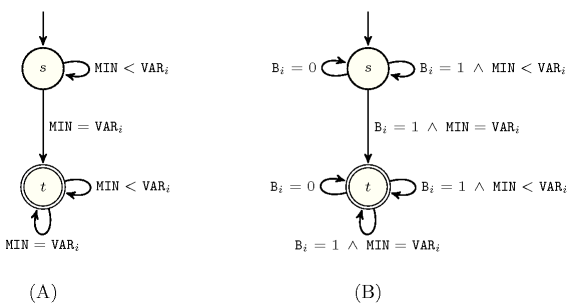### 3.7.172. Open automaton constraint

A constraint for which the set of solutions can be recognised by a so called open automaton. An open automaton is a finite deterministic automaton taking as input a sequence of variables ${V}_{1}{V}_{2}\cdots {V}_{n}$ as well as a sequence of 0-1 variables ${B}_{1}{B}_{2}\cdots {B}_{n}$. A variable ${B}_{i}$ $\left(1\le i\le n\right)$ set to value 0 means that the corresponding variable ${V}_{i}$ is removed from the sequence of variables ${V}_{1}{V}_{2}\cdots {V}_{n}$.

Consider a constraint $𝒞$ for which we already have a finite deterministic automaton $𝒜$ that only accepts the set of solutions of $𝒞$. Constructing the finite deterministic automaton ${𝒜}^{\text{'}}$ that only recognises the set of solutions to the open version of constraint $C$ can be done in a systematic way from the automaton $𝒜$. First, to each transition of $𝒜$ we add the fact that the corresponding Boolean variable must also be equal to 1. Second, to each state of $𝒜$ we add a loop transition for which the corresponding Boolean variable ${B}_{i}$ $\left(1\le i\le n\right)$ must be equal to 0 (since variable ${V}_{i}$ is ignored, we stay within the same state). Figure 3.7.45 illustrates this construction in the context of the $\mathrm{𝚖𝚒𝚗𝚒𝚖𝚞𝚖}$ constraint and of its open counterpart, the $\mathrm{𝚘𝚙𝚎𝚗}_\mathrm{𝚖𝚒𝚗𝚒𝚖𝚞𝚖}$ constraint.

##### Figure 3.7.45. Constructing the (B) automaton of the $\mathrm{𝚘𝚙𝚎𝚗}_\mathrm{𝚖𝚒𝚗𝚒𝚖𝚞𝚖}$$\left(\mathrm{𝙼𝙸𝙽},〈{\mathrm{𝚅𝙰𝚁}}_{1}{𝙱}_{1},{\mathrm{𝚅𝙰𝚁}}_{2}{𝙱}_{2},\cdots ,{\mathrm{𝚅𝙰𝚁}}_{n}{𝙱}_{n}〉\right)$ constraint from the (A) automaton of the $\mathrm{𝚖𝚒𝚗𝚒𝚖𝚞𝚖}$$\left(\mathrm{𝙼𝙸𝙽},〈{\mathrm{𝚅𝙰𝚁}}_{1},{\mathrm{𝚅𝙰𝚁}}_{2},\cdots ,{\mathrm{𝚅𝙰𝚁}}_{n}〉\right)$ constraint